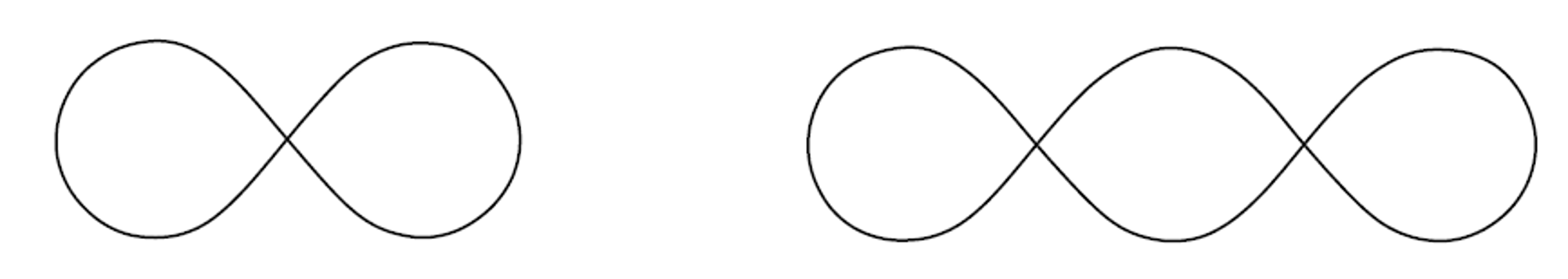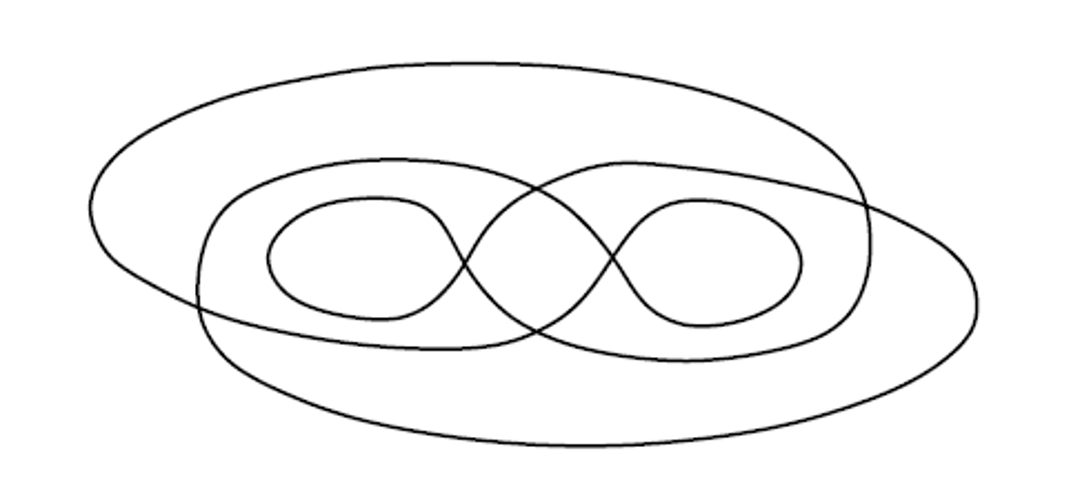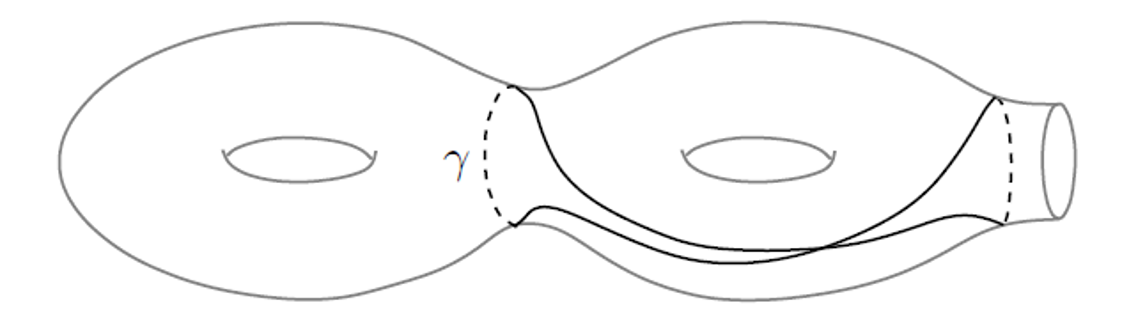# Farb and Friends

The Farb and Friends seminar is an informal seminar organized by the Farb working group for graduate students and postdocs to share expository talks in a low-stakes environment.

• When: Every Monday, 4pm CDT
• Where: Eckhart 312 (or Zoom)
• Contact: To join the mailing list, please email Soohyun Park or Peng Hui How

### Upcoming Talks

Title Speaker Date
Bounding immersed loops by immersed surfaces Which of the following immersed circles on the plane bound *immersed* disks? This turns out to be a complicated problem in general. What about the analogous problem of bounding immersed loops on a higher genus surface by immersed surfaces (see the last figure for an example)? A stable version of this problem turns out to have a clean answer, using hyperbolic geometry and a few related invariants (rotation number, stable commutator length). I'll explain this work of Calegari.Lvzhou Chen (UT Austin) 5/16
TBD Frederik Benirschke 5/23

### Past Talks

Spring 2022
Title Speaker Date
Etale cohomology I have been blackboxing etale cohomology for a long time, simply thinking of it as singular cohomology with a Galois action. In this talk, I will try to explain how etale cohomology is closely related to the Galois action. For example, the etale cohomology of a field is the same as its Galois cohomology. Then I would like to explore a bit why etale cohomology, in contrast to sheaf cohomology on Zariski sites, yields the correct Betti numbers by examples. I would say a few words about Galois representations coming from etale cohomology.
Chengyang Bao 5/9
A boring dynamical system and why it's so interesting A couple years ago I noticed an interesting way to build a dynamical system on the space of unordered configurations of 3 points in the plane. To my chagrin, this turned out to be a boring dynamical system. However, it's boring for a very interesting reason! In this talk you'll get configuration spaces, elliptic curves in pencils, tetrahedra, torsion, and more. This talk may not be for everyone, but it may be just right for you.
Trevor Hyde 5/2
Boundaries of Groups and Cohomology There are several notions of boundaries of groups. For instance, given a Gromov hyperbolic group, one can define the Gromov boundary as equivalence classes of geodesic rays. I will explain a theorem of Bestvina and Mess relating the cohomology of this space to the cohomology of the group. Another notion of boundary is the Poisson boundary, which arises from probability theory. If there is time, I will try to convince you that this boundary should also give us cohomological information.
Oliver Wang 4/25
The Simpson Twistor In this talk, I'll present a perspective on Hodge theory due to Simpson. It turns out there is a certain scheme over R, the twistor curve, that encodes much of the information relevant to Hodge theory. Topologically, this curve looks like the real projective plane. I'll explain an equivalence of categories between pure real Hodge structures of weight n and U(1)-equivariant vector bundles of slope n/2. Further, it turns out that forgetting the U(1)-action relates to an interesting category of real isocrystals which in odd weights is equivalent to quaternionic vector spaces. If time permits, I'll mention (without getting into technical details) some fascinating similarities and differences with the p-adic setting, which is how I got interested in this topic.
Gal Porat 4/18
Approximate subgroups in Lie groups We will discuss the notion of approximate groups in Lie groups and their relation to nonperiodic tilings of $$mathbb{R}^n$$ or hyperbolic space and discuss recent results of Simon Machado regarding approximate subgroups in Lie groups of higher rank.
Quartic curves and K3 surfaces I will talk about my recent work regarding the computation of the monodromy of the family of K3 surfaces branching over smooth quartic curves. I hope to elucidate the beautiful interplay between many objects, including:
• 27 lines on a cubic surface
• 28 bitangents to a quartic curve
• Degree 2 del Pezzo surfaces
• Weyl groups and graph automorphisms
• Hodge and cyclic decompositions
• Lattices and gluing groups
• Moduli spaces and framings
• The Torelli theorem for K3 surfaces
Adán Medrano Martín Del Campo 4/4

Winter 2022
Title Speaker Date
On homological stability for discriminant complements and related spaces I will survey homological stability for discriminant complements, discussing some results and open questions.
Ishan Banerjee 3/7
Peg Problem

Given a Jordan curve on the plane, can you always find 4 points that they form a rectangle, or even a square? What about other shapes? What about an imbedded 2 sphere in $$\mathbb{R}^3$$, can you find similar statement? This presents work of Cole Hugelmeyer, Josh Greene and Andrew Lobb.

Lei Chen (UMaryland) 2/28
Orthoschemes

I will talk about a forgotten theorem of Coxeter, which gives a generalization of Gauss’s Pentogramma Mirificum. I will explain its role in the Goncharov Program and say a few words about its role in the proof of the theorem of Böhm on volumes of hyperbolic polytopes.

Daniil Rudenko 2/21
Invariant random subgroups and asymptotic invariants

Let $$M$$ be a closed aspherical manifold with the fundamental group $$\Gamma$$. Suppose we are interested in the growth of topological invariants of a sequence of finite covers $$M_n$$ of $$M$$. These can be often read from the limit objects which are constructed using the sequence $$M_n$$. One way of doing it is by considering the weak-star limits of normalized counting measures supported on the conjugates of the fundamental group of $$M_n$$ inside the space of subgroups of $$\Gamma$$. The limit is a random subgroup whose distribution is invariant by conjugation by $$\Gamma$$ - we call them "invariant random subgroups”. I my talk I will explain how they can be applied to various problems in geometry and how they fit in the more general framework of unimodular measures on moduli spaces.

Recording   Notes
Mikolaj Fraczyk 2/14
Iterated monodromy groups and finite automata

Given a rational function f(x) with complex coefficients, the iterates of f(x) determine a sequence of finite degree branched covers of the sphere. Finite branched covers of the sphere are essentially determined by their monodromy, so it stands to reason that the dynamics of f(x) should be encoded in this sequence of monodromy groups. But how exactly does that work? In this talk I'll introduce the notion of an iterated monodromy group, show how this leads us by the nose to the important concept of a post-critically finite map, and see how this relates to finite automata acting on infinite trees.

Trevor Hyde 2/7
Sextic curves with six cusps

There are two kinds of sextic curves C in P^2 with exactly six ordinary cusps and no other singularities: one kind has $$\pi_1(\mathbb{P}^2 - C) = \mathbb{Z}/2 * \mathbb{Z}/3$$ non-abelian, and the other has $$\pi_1(\mathbb{P}^2 - C) = \mathbb{Z}/6$$ abelian. These two kinds of curves are distinguished by whether or not the six cusp points lie on a conic. This is very weird and has confused me for a long time. I'm going to talk about these sextics, some cool things, some weird things, and some things that still confuse me about all this.

Peter Huxford 1/31
Polynomial equations in groups

I will discuss a notion of polynomial equations in groups motivated by a question about enlarging a group by adding a generator. Then I will explain why unitary groups are "algebraically closed".

Recording
Nate Harman (UMich) 1/24

Fall 2021
Title Speaker Date
Prym varieties

The Prym construction associates a principally polarized abelian variety of dimension $$g-1$$, referred to as the Prym variety of the cover, to a double cover $$f: Y \to X$$ of a smooth genus $$g$$ curve. I will discuss some interesting properties of this construction.

Carlos Marcelo Servan 12/6
$$\text{Out}(S_6)$$ and Pascal's Mysticum

I'll discuss a fun (and the historically original) appearance of an outer automorphism of $$S_6$$ by fussing around with hexagons on conic plane curves. Along the way we meet up with Steiner, Plücker, Salmon, Kirkman, Cayley, Bacharach, Braikenridge, Maclaurin, and of course Pascal.

Michael Klug 11/29
Involutions in the plane Cremona group

The plane Cremona group $$\text{Cr}(2)$$ (over $$\mathbb C$$) is the group of birational automorphisms of $$\mathbb{CP}^2$$. There is a classification of the conjugacy classes of order $$2$$ elements of $$\text{Cr}(2)$$ into three types: de Jonquières, Geiser, and Bertini. In this talk, I will describe these three types of involutions explicitly.

Seraphina Lee 11/15
Solving Equations with Dynamics

It is well-known that there is no general solution by radicals for polynomials of degree at least 5...but are there other ways to solve polynomial equations? In this talk I will discuss the possibility of finding polynomial roots through iteration of rational functions. We will see, following McMullen, that iteration allows us to solve the general quintic (and not much else!)

Trevor Hyde 11/8
Mednykh's Formula

Mednykh's formula counts homomorphisms from the fundamental group of a surface to a finite group $$G$$ in terms of the representation theory of $$G$$. We'll discuss two proofs of this formula, as well as related questions.

Joshua Mundinger 11/1
Mapping class groups of connected sums of $$S^2 \times S^1$$

Recently Bruno Zimmermann posted a short paper on the Nielsen realization problem for the mapping class groups of the three-manifolds formed by taking finite connected sums of $$S^2 \times S^1$$. This made me curious about what is known about these groups! I will survey some of what I found out and will also discuss some of the results and arguments in Zimmermann’s paper.

Justin Lanier 10/25
Linear subspaces on a Fano $$k$$-plane invariant class of varieties

Consider the following statement: "The space of $$k$$-planes contained in varieties in $$A$$ is in $$A$$." What can $$A$$ be? For a certain $$A$$, this will depend on a special property of varieties represented by points of $$A$$.

Soohyun Park 10/18
What's so special about the homology of algebraic varieties?

It turns out that the (co)homology groups of an algebraic variety admit a number of amazing structures, which go beyond the homology of ordinary topological spaces. I will talk about several of these structures, how they reflect the "rigidity" of algebraic geometry, and about several deep analogies/conjectures connecting them.

Phil Tosteson 10/11

Spring 2021
Title Speaker Date
Groups acting on graphs

Group actions on graphs reveal information about the group itself. We demonstrate this on SL(2,Z) and its various generalizations. For example, we show that mapping class groups of surfaces with positive genus is finitely generated by Dehn twists.

Recording   Notes
Peng Hui How 6/7
Stable torsion length

The stable torsion length in a group is the stable word length with respect to the set of all torsion elements. We show that the stable torsion length vanishes in crystallographic groups. We then give a linear programming algorithm to compute a lower bound for stable torsion length in free products of groups. Moreover, we obtain an algorithm that exactly computes stable torsion length in free products of finite abelian groups. The nature of the algorithm shows that stable torsion length is rational in this case. As applications, we give the first exact computations of stable torsion length for nontrivial examples. (Joint work with Lvzhou Chen.)

Chloe Avery 5/31
Every surface is a leaf

Every closed 3-manifold admits foliations, where the leaves are surfaces. For a given 3-manifold, which surfaces can appear as leaves? Kerékjártó and Richards gave a classification up to homeomorphism of noncompact surfaces, which includes surfaces with infinite genus and infinitely many punctures. In their 1985 paper "Every surface is a leaf", Cantwell–Conlon prove that for every orientable noncompact surface L and every closed 3-manifold M, M has a foliation where L appears as a leaf. We will discuss their paper and construction and the surrounding context.

Recording   Notes
Justin Lanier 5/24
On the Torelli theorems

I will give an overview of the Torelli theorems for curves and K3 surfaces.

Recording
Adán Medrano Martín Del Campo 5/17
Platonic solids and Kleinian singularities

A remarkably long list of mathematical structures can be classified by the simply laced Dynkin diagrams, i.e. those of types A, D and E (but not B or C). I will discuss just some of the connections I’ve learned between different structures sharing these classifications.

Recording
Peter Huxford 5/10
Reflections and indefinite orthogonal groups

Consider the groups O(f, \mathbb{Z}) of integral matrices preserving an integral quadratic form f of signature (n, 1) for some n \geq 2. Such a group is called reflexive if its subgroup generated by reflections has finite index. In 1972, Vinberg proposed an algorithm to determine the reflexivity of these groups and applied it to the case n \leq 17. In this talk, we will give an overview of some basics of reflection groups and Vinberg's algorithm.

Recording
Seraphina Lee 5/3
A strange state of affairs in P^2

We will discuss a combinatorial plane geometry question that is currently best understood using a rather deep result on properties of algebraic surfaces.

Recording
Soohyun Park 4/26
Configuration spaces with summable labels

The nth configuration space of a manifold M can be thought of as the space parameterizing n unlabelled particles in M, such that the particles are not allowed to collide with each other. Configuration spaces with summable labels are variants of configuration spaces where the particles now have names, and there is a prescribed rule for what happens when particles of different types collide (e.g. when a muon and a gluon collide they turn into a pion). Often, important spaces in algebraic topology are secretly configuration spaces with summable labels! I will talk about examples involving classifying spaces and mapping spaces, the Dold-Thom theorem and its generalizations.

Recording   Notes
Phil Tosteson 4/19
A crash course in Tannakian formalism

Just how much information does the representation theory of a group carry about the group itself? Well that depends what you mean by "representation theory" or "group"...

Recording
Nate Harman 4/12

Winter 2021
Title Speaker Date
Quasi-morphism and uniformly perfect groups

What does the Dedekind eta function and the Hirzebruch signature defect have to do with each other? A priori, nothing much. But thanks to the fact that PSL(2,R) and SL(2,R) both act on the circle, the defect of log(eta) and the signature defect of the mapping torus pretty much differ by a constant factor, thanks to a link granted by the Euler class of Homeo^+(S^1).

Recording   Notes
Peng Hui How 3/15
Stable birational equivalence vs. cut and paste relations

We will focus on a result of Larsen--Lunts that gives a close connection between stable birational equivalence classes of smooth projective varieties and cut and paste relations. Following some background and a sketch of a proof, we will give applications to the overall structure of cut and paste relations and specific independence results.

Recording
Soohyun Park 3/8
Finite group actions on 4-manifolds

Suppose a finite, cyclic group G acts on a 4-manifold. What can we say about the fixed set of this action? In this talk, I will discuss some conditions one can impose on the action (e.g. preserving the orientation or the spin structure) and their consequences on the topology of the fixed set.

Recording   Notes
Seraphina Lee 3/1
Polygonal complexes

Polyhedral complexes are 2-dimensional simplicial complexes which are created by gluing together regular Euclidean k-gons along their edges. A natural question is how many (simply connected) polyhedral complexes exist which satisfy some collection of local gluing criteria, up to isomorphism. This talk is an introduction to polyhedral complexes, and gives an overview of some work that has been done in this direction, specifically when the polyhedral complexes are (k,L)-complexes.

Recording
Chloe Avery 2/22
Monodromy of families of hyperelliptic curves

We give a brief overview of the first sections of McMullen's paper Braid Groups and Hodge Theory, exploring unitary representations of the braid group given by the monodromy of a family of hyperelliptic curves.

Recording
Adán Medrano Martin Del Campo 2/15
Line bundles, divisors, and linear systems

We will explore the relationship between some of the fundamental objects of algebraic geometry, divisors and line bundles, from a concrete, geometric perspective, using the language of Čech cohomology.

Recording
Nat Mayer 2/8
Fundamental group of the complement of a plane algebraic curve

To better understand how a variety in affine or projective space "sits" in space, one can try to compute topological invariants of its complement. Such complements sometimes also parametrize the 'nice' or 'smooth' objects in a moduli space. I will discuss a method to compute the fundamental group of the complement of an algebraic curve (over ℂ) in the plane, and explicitly compute some examples. This is based on work originally due to Zariski and Van-Kampen, but the tools used, such as braid monodromy, are also useful in more complicated settings.

Recording
Peter Huxford 2/1
Group cohomology
Ronno Das 1/25
Spectral sequences Recording